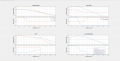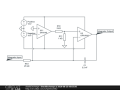# Practical implementation of a Dual op-amp integrator

#### Sambeet

Joined Feb 13, 2021
5
I found this circuit here on the web. The post claims of improving the open-loop gain of the op-amp by huge margins, which is true. But if we try to analyse this circuit in MATLAB, we find that this has zero phase margin at cross-over, which gives gain peaking. My question is, can we use this circuit for practical usage as it appears to be very close to instability?#### crutschow

Joined Mar 14, 2008
32,031
Why do you want such a high open-loop gain?
Most op amps have a high open-loop gain that increasing would have no significant effect on the integrator accuracy.

#### Sambeet

Joined Feb 13, 2021
5
It is because I want a high bandwidth opamp like THS4631, but the open-loop gain of that is about 10000. With such a low open loop gain, the low cut off frequency is not enough for my application. So I need to increase my open-loop gain for getting a lower cut-off frequency.

#### crutschow

Joined Mar 14, 2008
32,031

#### Sambeet

Joined Feb 13, 2021
5
The circuit is an integrator for PCB Rogowski Coil. With Ri =470 and Ci=1nF, the low-cutoff frequency comes out to be 33Hz. The Ri and Ci are fixed with respect to the application as that also decides the sensitivity. So I want to increase the Aol by a factor of 20, to get a lower cut-off frequency.

#### crutschow

Joined Mar 14, 2008
32,031
If the integrator bode gain/phase plot looks normal, then I would think you could use the two op amps in series as shown without a stability problem.

#### Sambeet

Joined Feb 13, 2021
5
Let me reframe the question. The bode plot shows zero phase margin, but the crossover is at 200MHz.I want to use it till a frequency of 30MHz though (it shows proper gain phase characteristics till that frequency). So the question is can I use this integrator like this, i.e. though the system is unstable, can the use be limited to before crossover for operation?

#### LvW

Joined Jun 13, 2013
1,644
The circuit is an integrator for PCB Rogowski Coil. With Ri =470 and Ci=1nF, the low-cutoff frequency comes out to be 33Hz. The Ri and Ci are fixed with respect to the application as that also decides the sensitivity. So I want to increase the Aol by a factor of 20, to get a lower cut-off frequency.
I found this circuit here on the web. The post claims of improving the open-loop gain of the op-amp by huge margins, which is true. But if we try to analyse this circuit in MATLAB, we find that this has zero phase margin at cross-over, which gives gain peaking. My question is, can we use this circuit for practical usage as it appears to be very close to instability?
However, there are many other topologies for "composite" amplifiers (consisting of two opamps) with other stability properties.
Example: S. Michael, W.B. Mikhael, "Inverting intergator and active filter applications of composite opamps" (IEEE Trans., Vol. CAS-34. No 5).

As an alternative, you can improve the stability margin using external phase compensation (suitable R-C-combination across the input terminals of the first opamp). This method leads to a continuous increase of 1/beta (inverse feedback factor) and enables a zero (change to a horizontal line) before 1/beta crosses the open-loop amplifier gain magnitude.

Last edited:
•Sambeet